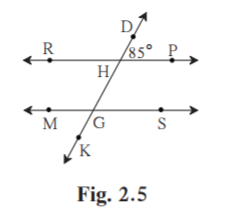No products in the cart.

# 1.in figure2.5 lineRP||line ms and line …## CBSE, JEE, NEET, CUET

Question Bank, Mock Tests, Exam Papers

NCERT Solutions, Sample Papers, Notes, Videos

1.in figure2.5 lineRP||line ms and line DK is their transversal dhp=85° find the measures of following angles (I) RHD (ii) PHG (iii) HGS (IV)MGK

Gaurav Seth 2 years, 7 months ago

1. In figure 2.5, line RP || line MS and line DK is their transversal. DHP = 85° Find the measures of following angles.

(i) RHD (ii) PHG (iii) HGS (iv) MGKSolution:

Given RPMS.

DK is the transversal.

(i) DHP = 85˚

RHD = 180-85 = 95˚ [Linear pair]

(ii) PHG = RHD = 95˚ [RHD and PHG are vertically opposite angles]

(iii)HGS = 180-95 = 85˚ [PHG and HGS are corresponding interior angles]

(iv)MGK = HGS = 85˚ [Vertically opposite angles]

Related Questions## myCBSEguide

Trusted by 1 Crore+ Students## Test Generator

Create papers online. It's FREE.## CUET Mock Tests

75,000+ questions to practice only on myCBSEguide app